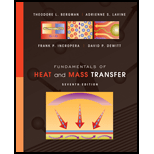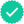Fundamentals of Heat and Mass Transfer
7th Edition
ISBN: 9780470501979
Author: Frank P. Incropera, David P. DeWitt, Theodore L. Bergman, Adrienne S. Lavine
Publisher: Wiley, John & Sons, Incorporated#### Videos

Textbook QuestionChapter 1, Problem 1.1P

The thermal conductivity of a sheet of rigid, extrudedinsulationis reported to be k = 0.029 W/m K . Themeasured temperature difference across a 20-mm-thicksheet of the material is T 1 T 2 = 10 ° C .
(a) What is the heat flux through a 2 m × 2 m sheet ofthe insulation?
(b) What is the rate of heat transfer through the sheet of insulation?

(a)

Expert SolutionTo determine

Heat Flux through a Sheet of insulation of dimension 2 m×2 m .

Heat Flux through a Sheet of insulation of dimension 2 m×2 m is 14.5 W/m2 .

### Explanation of Solution

Given:

Thermal conductivity of insulation is 0.029W/mK .

Thickness of Sheet is 20 mm .

Temperature difference through sheet is 10°C .

Dimension of sheet is 2m×2m .

Concept used:

Write the expression for heat flux passing through the sheet of insulation.

QA=kΔTΔx ........ (1)

Here, Q is the rate of heat transfer, A is the cross-sectional area of sheet, k is thermal conductivity of specimen, ΔT is the temperature difference through the sheet and Δx is the thickness of sheet.

Calculation:

Substitute 10°C for ΔT , 0.029W/mK for k and 20mm for Δx in equation (1).

QA=(0.029)10( 20 mm( 1 m 1000mm ))=14.5 W/m2

Conclusion:

Thus, heat Flux through a Sheet of insulation of dimension 2 m×2 m is 14.5 W/m2 .

(b)

Expert SolutionTo determine

Rate of heat transfer through sheet of insulation.

Rate of heat transfer through sheet of insulation is 58W .

### Explanation of Solution

Concept used:

Calculate the area of rectangular sheet as follows:

A=2 m×2 m=4 m2

Write the expression for rate of heat transfer.

Q=kAΔTΔx ........ (2)

Calculation:

Substitute 10°C for ΔT , 0.029W/mK for k , 4m2 for A and 20mm for Δx in equation (1).

Q=(4)(0.029)10( 20 mm( 1 m 1000mm ))=58W

Conclusion:

Thus, rate of heat transfer through sheet of insulation is 58W .

### Want to see more full solutions like this?

Subscribe now to access step-by-step solutions to millions of textbook problems written by subject matter experts!
02:38
Students have asked these similar questions
Why did you divide by 2 the thermal conductivity of E,F,B and C? Why not use parallel resistance calculation instead?
In a thermal power plant, a horizontal copper pipe of "D" diameter, "L" length and thickness 1.2 cm enters into the boiler that has the thermal conductivity as 0.37 W/mK. The boiler is maintained at 113￼C and temperature of the water that flows inside the pipe is at 29￼C. If the energy transfer (Q) is 118779 kJ in 7 hours. Calculate: 4-Length of the pipe, if D = 0.017 L.  5-Pipe Diameter (in mm)
A 50-cm-diameter pipeline in the Arctic carries hot oil at 30◦C and is exposed to a surrounding temperature of −20◦C. A special powder insulation 5 cm thick surrounds the pipe and has a thermal conductivity of 7 mW/m · ◦C. The convection heat-transfer coefficient on the outside of the pipe is 9 W/m2 · ◦C. Estimate the energy loss from the pipe per meter of length.   (Please give step-by-step solution)